# NCERT Solutions for Class 12 Maths Chapter 5: Continuity and Differentiability

In this page we have NCERT Solutions for Class 12 Maths Chapter 5: Continuity and Differentiability for EXERCISE 5.1 . Hope you like them and do not forget to like , social share and comment at the end of the page.

Main Idea of the Exercise
A function is continuous at x = c if the function is defined at x = c and if the value of the function at x = c equals the limit of the function at x = c. If f is not continuous at c, we say f is discontinuous at c and c is called a point of discontinuity of f

Question 1
Prove that the function f(x) = 5x – 3 is continuous at x = 0, at x = – 3 and at x = 5.
Solution
At x=0
f (0) = 5(0) – 3=-3
$\lim_{x \rightarrow 0} f(x)=\lim_{x \rightarrow 0} (5x-3)=5(0) -3=-3$
Therefore, limit and f (0) are same. So, it is continuous
At x=-3
f(-3) = 5(-3) – 3=-18
$\lim_{x \rightarrow -3} f(x)=\lim_{x \rightarrow -3} (5x-3)=5(-3) -3=-18$
Therefore, limit and f (-3) are same. So, it is continuous
At x=5
f(5) = 5(5) – 3=22
$\lim_{x \rightarrow 5} f(x)=\lim_{x \rightarrow 5} (5x-3)=5(5) -3=22$
Therefore limit and f(5) are same. So, it is continuous

Question 2.
Examine the continuity of the function f(x) = 2x2 – 1 at x = 3.
Solution
At x=3
f(3) = 2x2 – 1 =2(9) – 1=17
$\lim_{x \rightarrow 3} f(x)=\lim_{x \rightarrow 3} (2x^2 -1)=2(9) -1=17$ Therefore limit and f(3) are same. So, it is continuous at x=3

Question 3.
Examine the following functions for continuity.
(a) f (x) = x – 5
(b) f (x) =1/(x-5) , x ≠5
(c) f (x) = (x2 -25)/ (x+5), x ≠-5
(d) f (x) = | x – 5|
Solution
a) f (x) = x – 5
The function is clearly defined at every point and f (c) =c-5 for every real number c.
Also,
$\lim_{x \rightarrow c} f(x)=\lim_{x \rightarrow c} (x-5)=c-5$
Since
$f(c) =\lim_{x \rightarrow c} f(x)$
So, function is continuous at every real number
b) f (x) =1/(x-5)  , x ≠5
The function is clearly defined at every point except x=5 and f (c) =1/(c-5) for every real number c except c=5
$\lim_{x \rightarrow c} f(x)=\lim_{x \rightarrow c} \frac {1}{x-5}=\frac {1}{c-5}$
Since
$f(c) =\lim_{x \rightarrow c} f(x)$
So, function is continuous at every real number in its domain
c) f (x) = (x2 -25)/ (x+5), x ≠-5
The function is clearly defined at every point except x=-5 and f (c) = (c2 -25)/ (c+5)= c-5, for every real number c except c=-5
$\lim_{x \rightarrow c} f(x)=\lim_{x \rightarrow c} \frac {x^2-25}{x+5}=\frac {c^2-25}{c+5}=c-5$ Since
$f(c) =\lim_{x \rightarrow c} f(x)$
So, function is continuous at every real number in its domain
d)  f (x) = | x – 5|
This function can re-written as
f(x)=\left\{\begin{matrix} 5-x, & x <5 \\ x- 5, & x \geq 5 \end{matrix}\right. This function f is defined at all points of the real line.
For continuity in these type of question, we must look for the continuity at the common point and point outside the common point
Let c be a point on a real line. Then, c < 5 or c = 5 or c > 5
Case 1 c < 5
f (x) = 5 – x
f (c) = 5 – c
$\lim_{x \rightarrow c} f(x)=\lim_{x \rightarrow c} (5 -x)=5-c$
Since
$f(c) =\lim_{x \rightarrow c} f(x)$
Function is continuous at   c< 5
Case 2 c > 5
f (x) = x – 5
f (c) = c – 5
$\lim_{x \rightarrow c} f(x)=\lim_{x \rightarrow c} (x -5)=c-5$
Since
$f(c) =\lim_{x \rightarrow c} f(x)$
Function is continuous at   c > 5
Case 3 c = 5
f (x) = x – 5
f (5) = 5 – 5=0
$\lim_{x \rightarrow 5^-} f(x)=\lim_{x \rightarrow 5^-} (5 -x)=5-5=0$
$\lim_{x \rightarrow 5^+} f(x)=\lim_{x \rightarrow 5^+} (x -5)=5-5=0$
Since
Function is continuous at   c =5
Hence, f is continuous at every real number and therefore, it is a continuous function.

Question 4.
Prove that the function f (x) = xn is continuous at x = n, where n is a positive integer.
Solution
The given function is f (x) = xn
It is evident that f is defined at all positive integers, n, and its value at n is nn
$\lim_{x \rightarrow n} f(x)=\lim_{x \rightarrow n} x^n=n^n$
Therefore, f is continuous at n, where n is a positive integer.

Question 5.
Is the function f defined by?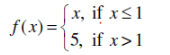continuous at x = 0? At x = 1? At x = 2?
Solution
At x = 0,
f(0) = 0.
$\lim_{x \rightarrow 0} f(x)=\lim_{x \rightarrow 0} x=0$
Therefore, f is continuous at x = 0
At x = 1,
f(1) = 1.
The left-hand limit of f at x = 1 is,
$\lim_{x \rightarrow 1^-} f(x)=\lim_{x \rightarrow 1^-} x=1$
The right-hand limit of  f at x = 1 is,
$\lim_{x \rightarrow 1^+} f(x)=\lim_{x \rightarrow 1^+} x=5$
Therefore, function is not continuous at x=1
At x = 2,
f(2) = 5.
$\lim_{x \rightarrow 2} f(x)=\lim_{x \rightarrow 2} 5=5$
Therefore, f is continuous at x = 2

### Find all points of discontinuity of f, where f is defined by

Question 6.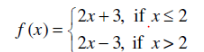Solution
This function f is defined at all points of the real line.
For continuity in these type of question, we must look for the continuity at the common point and point outside the common point
Let c be a point on a real line. Then, c < 2 or c = 2 or c > 2
It can be easily proved for c < 2 and c> 2. We have seen that in lot of previous example
For c=2
f(2) =2(2)+3=7
The left-hand limit of f at x = 2 is,
$\lim_{x \rightarrow 2^-} f(x)=\lim_{x \rightarrow 2^-} (2x+3)=7$
The right-hand limit of f at x = 2 is,
$\lim_{x \rightarrow 2^+} f(x)=\lim_{x \rightarrow 2^+} (2x-3)=1$
It is observed that the left and right hand limit of f at x = 2 do not coincide.
Therefore, f is not continuous at x = 2
Hence, x = 2 is the only point of discontinuity of f.

Question 7.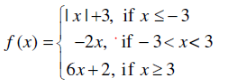Solution
For   x≤ -3
F(x) = |x| +3
Since x is negative in that, we can re-write this as
F(x) = 3-x
This function f is defined at all points of the real line.
For continuity in these type of question, we must look for the continuity at the common point and point outside the common point
Let c be a point on a real line. Then, c < -3 or c = -3 or -3 < c  < 3 or c=3 or c> 3
It can be easily proved for c < -3 , -3 <  c  < 3 And c> 3  We have seen that in lot of previous example, we will look at common points
For c=-3
f(-3) =3-(-3) =6
The left-hand limit of f at x = -3 is,
$\lim_{x \rightarrow -3^-} f(x)=\lim_{x \rightarrow -3^-} (3-x)=6$
The right-hand limit of f at x = -3 is,
$\lim_{x \rightarrow -3^+} f(x)=\lim_{x \rightarrow -3^+} (-2x)=6$
Therefore, f is continuous at x = -3
For c=3
f(3) =6(3)+2 =20
The left-hand limit of f at x = 3 is,
$\lim_{x \rightarrow 3^-} f(x)=\lim_{x \rightarrow 3^-} (-2x)=-6$
The right-hand limit of f at x = 3 is,
$\lim_{x \rightarrow 3^+} f(x)=\lim_{x \rightarrow 3^+} (6x+2)=20$
It is observed that the left and right hand limit of f at x = 3 do not coincide.
Therefore, f is not continuous at x = 3
Hence, x = 3 is the only point of discontinuity of f.

Question 8.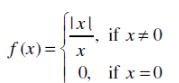Solution
We know for x < 0 , |x|=-x  and x> 0, |x|=x, So this function can be written as
$f(x)=\begin{cases} & \frac {|x|}{x} =\frac {|-x}{x}=-1, \text{ if } \; x < 0 \\ & 0, \text{ if } \; x = 0 \\ & \frac {|x|}{x} =\frac {|x}{x}=1,\text{ if } \; x > 0 \end{cases}$
This function f is defined at all points of the real line.
For continuity in these type of question, we must look for the continuity at the common point and point outside the common point
Let c be a point on a real line. Then, c < 0 or c = 0 or  c> 0
It can be easily proved for c < 0 And c> 0  We have seen that in lot of previous example, we will look at common points
For c=0
F(0) =0
The left-hand limit of f at x = 0 is,
$\lim_{x \rightarrow 0^-} f(x)=\lim_{x \rightarrow 0^-} (-1)=-1$
The right-hand limit of f at x = 0 is,
$\lim_{x \rightarrow 0^+} f(x)=\lim_{x \rightarrow 0^+} (1)=1$
It is observed that the left and right hand limit of f at x = 0 do not coincide.
Therefore, f is not continuous at x = 0
Hence, x = 0 is the only point of discontinuity of f.

Question 9.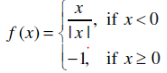Solution
We know for x <  0 , |x|=-x  So this function can be written as
F(x) =-1 if x < 0
And
F(x) =-1 if x≥0
This function f is defined at all points of the real line.
For continuity in these type of question, we must look for the continuity at the common point and point outside the common point
Let c be a point on a real line. Then, c < 0 or c = 0 or  c> 0
It can be easily proved for c < 0 And c> 0 . We have seen that in lot of previous example, we will look at common points
For c=0
F(0) =0
The left-hand limit of f at x = 0 is,
$\lim_{x \rightarrow 0^-} f(x)=\lim_{x \rightarrow 0^-} (-1)=-1$
The right-hand limit of f at x = 0 is,
$\lim_{x \rightarrow 0^+} f(x)=\lim_{x \rightarrow 0^+} (-1)=-1$
Therefore, f is continuous at x = 0
Hence, there is no point of discontinuity of  f.

Question 10.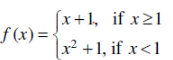Solution
This function f is defined at all points of the real line.
For continuity in these type of question, we must look for the continuity at the common point and point outside the common point
Let c be a point on a real line. Then, c < 1 or c = 1 or c> 1
It can be easily proved for c < 1 And c> 1 .We have seen that in lot of previous example, we will look at common points
For c=1
f(1) =1+1=2
The left-hand limit of f at x = 1 is,
$\lim_{x \rightarrow 1^-} f(x)=\lim_{x \rightarrow 1^-} (x^2 +1)=2$
The right-hand limit of f at x = 1 is,
$\lim_{x \rightarrow 1^+} f(x)=\lim_{x \rightarrow 1^+} (x +1)=2$
Therefore, f is continuous at x = 1
Hence, there is no point of discontinuity of f.

Question 11.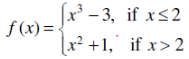Solution
This function f is defined at all points of the real line.
For continuity in these type of question, we must look for the continuity at the common point and point outside the common point
Let c be a point on a real line. Then, c < 2 or c = 2 or  c> 2
It can be easily proved for c < 2 And c> 2  .We have seen that in lot of previous example, we will look at common points
For c=2
f(1) =x3 -3 =8-3=5
The left-hand limit of f at x = 2 is,
$\lim_{x \rightarrow 2^-} f(x)=\lim_{x \rightarrow 2^-} (x^3 -5)=5$
The right-hand limit of f at x = 2 is,
$\lim_{x \rightarrow 2^+} f(x)=\lim_{x \rightarrow 2^+} (x^2 +1)=5$
Therefore, f is continuous at x = 2
Hence, there is no point of discontinuity of f.

Question 12.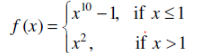Solution
This function f is defined at all points of the real line.
For continuity in these type of question, we must look for the continuity at the common point and point outside the common point
Let c be a point on a real line. Then, c < 1 or c = 1 or  c> 1
It can be easily proved for c < 1 And c> 1 . We have seen that in lot of previous example, we will look at common points
For c=1
F(1) =x10 -1 =0
The left-hand limit of f at x = 1 is,
$\lim_{x \rightarrow 1^-} f(x)=\lim_{x \rightarrow 1^-} (x^{10} -1)=0$
The right-hand limit of f at x = 1 is,
$\lim_{x \rightarrow 1^+} f(x)=\lim_{x \rightarrow 1^} (x^2)=1$
Therefore, f is discontinuous at x = 1
Hence, x = 1 is the only point of discontinuity of f.

Question 13. Is the function defined by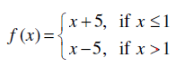a continuous function?
Solution
This function f is defined at all points of the real line.
For continuity in these type of question, we must look for the continuity at the common point and point outside the common point
Let c be a point on a real line. Then, c < 1 or c = 1 or   c> 1
It can be easily proved for c < 1 And c> 1.  We have seen that in lot of previous example, we will look at common points
For c=1
f(1) =x+5 =6
The left-hand limit of f at x = 1 is,
$\lim_{x \rightarrow 1^-} f(x)=\lim_{x \rightarrow 1^-} (x+5)=6$
The right-hand limit of f at x = 1 is,
$\lim_{x \rightarrow 1^+} f(x)=\lim_{x \rightarrow 1^} (x-5)=-4$
Therefore, f is discontinuous at x = 1
Hence, x = 1 is the only point of discontinuity of f.

### Discuss the continuity of the function f, where f is defined by

Question 14.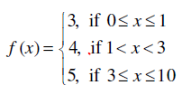Solution
The given function is defined at all points of the interval [0,10].
For continuity in these type of question, we must look for the continuity at the common point and point outside the common point
Let c be a point on a real line. Then, 0 ≤ c < 1 or c = 1 or   1<c < 3 or c=3 or  3 <c < 10
It can be easily proved for 0 ≤ c < 1   ,  1< c < 3 , 3 <c < 10  We have seen that in lot of previous example, we will look at common points
For c=1
f(1) = 3
The left-hand limit of   f at x = 1 is,
$\lim_{x \rightarrow 1^-} f(x)=\lim_{x \rightarrow 1^-} (3)=4$
The right-hand limit of  f at x = 1 is,
$\lim_{x \rightarrow 1^+} f(x)=\lim_{x \rightarrow 1^} (4)=-4$
Therefore, f is discontinuous at x = 1
For c=3
f(3)= 5
The left-hand limit of  f at x = 3 is,
$\lim_{x \rightarrow 3^-} f(x)=\lim_{x \rightarrow 3^-} (4)=4$
The right-hand limit of  f at x = 3 is,
$\lim_{x \rightarrow 3^+} f(x)=\lim_{x \rightarrow 3^+} (5)=5$
Therefore, f is discontinuous at x = 3
Hence, f is not continuous at x = 1 and x = 3

Question 15.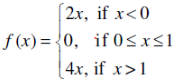Solution
This can be solved similarly above
Answer is f is not continuous only at x = 1

Question 16.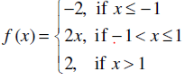Solution
This can be solved similarly above
Answer is f is continuous at all the points

Question 17.
Find the relationship between a and b so that the function f defined by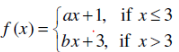is continuous at x = 3.
Solution
When the function is continuous at any point, it Left-hand limit, right-hand limit and function value are equal
$\lim_{x \rightarrow 3^+} f(x)=\lim_{x \rightarrow 3^-} f(x)=f(3)$
F(3) =3a+1
$\lim_{x \rightarrow 3^-} f(x)=\lim_{x \rightarrow 3^-} (ax+1) = 3a+1$
$\lim_{x \rightarrow 3^+} f(x)=\lim_{x \rightarrow 3^+} (bx+3) = 3b+3$
So
3a+1= 3a+1= 3b+3
Or a =b + (2/3)

Question 18.
For what value of λ is the function defined by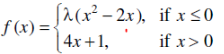continuous at x = 0? What about continuity at x = 1?
Solution
If f is continuous at x=0, then
$\lim_{x \rightarrow 0^+} f(x)=\lim_{x \rightarrow 0^-} f(x)=f(0)$
f(0) =0
$\lim_{x \rightarrow 0^-} f(x) =\lim_{x \rightarrow 0^-} \lambda(x^2 -2x) = 9$
$\lim_{x \rightarrow 0^+} f(x) =\lim_{x \rightarrow 0^+} \lambda(4x+1) = 1$
Since Left hand limit is not equal to right hand limit, Therefore, there is no value of λ for which the function f is continuous at x=0.
At x=1,
f(1)=4x+1=4×1+1=5
$\lim_{x \rightarrow 1} f(x) =\lim_{x \rightarrow 1}(4x+1) = 5$
Therefore, for any values of λ, f is continuous at x=1.

Question 19.
Show that the function defined by g(x) = x – [x] is discontinuous at all integral points. Here [x] denotes the greatest integer less than or equal to x.
Solution
This function g is defined at all integral points
Let n be an integer.
Then,
g(n)=n−[n]=n−n=0
Then left hand limit of f at x=n is
$\lim_{x \rightarrow n^-} f(x) =\lim_{x \rightarrow n^-}(x -[x]) =\lim_{x \rightarrow n^-} (x) - \lim_{x \rightarrow n^-} [x]=n -(n-1)=1$
Then right hand limit of f at x=n is,

$\lim_{x \rightarrow n^+} f(x) =\lim_{x \rightarrow n^+}(x -[x]) =\lim_{x \rightarrow n^+} (x) - \lim_{x \rightarrow n^+} [x]=n -n=0$
So, Left hand limit and Right hand limit are not equal
Therefore, f is not continuous at x=n
Hence, g is discontinuous at all integral points.

Question 20.
Is the function defined by f(x) = x2 – sin x + 5 continuous at x = π?
Solution
At x=π, f(x)=f(π)= π2−sinπ+5=π2 −0+5=π2+5
Consider
$\lim_{x \rightarrow \pi} f(x)= \lim_{x \rightarrow \pi} (x^2 - sin x + 5)$
Put x=π+h
If x→π, then it is evident that h→0
$\lim_{x \rightarrow \pi} f(x)= \lim_{x \rightarrow \pi} (x^2 - sin x + 5)=\lim_{h \rightarrow 0}[(\pi + h)^2 -sin (\pi + h) + 5]$
$=\lim_{h \rightarrow 0}(\pi + h)^2 - \lim_{h \rightarrow 0} sin (\pi + h) + \lim_{h \rightarrow 0} 5$
$=\lim_{h \rightarrow 0}(\pi + h)^2 - \lim_{h \rightarrow 0} (sin \pi cos h + cos \pi sin h) + \lim_{h \rightarrow 0} 5$
$=\pi^2 + 5$
So, function is continuous at x = π

Question 21.
Discuss the continuity of the following functions:
(a) f (x) = sin x + cos x
(b) f (x) = sin x – cos x
(c) f (x) = sin x . cos x
Solution
It is known that if g and h are two continuous functions, then g+ h , g − h ,g.h are also continuous functions
.So, It has to prove first that g (x) = sin x and h (x) = cos x are continuous functions.
Let g (x) = sin x
It is evident that g (x) = sin x is defined for every real number.
g(c) =sin(c)
Let c be a real number. Put x = c + h
If x → c, then h → 0
$\lim_{x \rightarrow c} g(x) =\lim_{x \rightarrow c} sinx$
$=\lim_{h \rightarrow 0} sin(c+h)=\lim_{h \rightarrow 0} [sin c cos h + cos c sin h]$
$=\lim_{h \rightarrow 0} sin c cos h + \lim_{h \rightarrow 0} cos c sin h= sin c + 0 =sin c$
So, g(x) is continuous function
Now
Let h (x) = cos x
It is evident that h (x) = cos x is defined for every real number.
h(c) =cos(c)
Let c be a real number. Put x = c + h
If x → c, then h → 0
$\lim_{x \rightarrow c} h(x) =\lim_{x \rightarrow c} cos x$
$=\lim_{h \rightarrow 0} cos(c+h)=\lim_{h \rightarrow 0} [cos c cos h - sin c sin h]$
$=\lim_{h \rightarrow 0} cos c cos h + \lim_{h \rightarrow 0} sin c sin h= cos c + 0 =cos c$
So, h(x) is a continuous function
Therefore, it can be concluded that
(a) f (x) = g (x) + h (x) = sin x + cos x is a continuous function
(b) f (x) = g (x) − h (x) = sin x − cos x is a continuous function
(c) f (x) = g (x) × h (x) = sin x × cos x is a continuous function

Question 22. Discuss the continuity of the cosine, cosecant, secant and cotangent functions.
Solution
Now these functions can be rewritten as
Cosec (x)   = 1/ sin (x)   sin(x) ≠0
Sec (x) = 1/cos (x)       cos (x) ≠0
Cot(x) = cos(x)/sin(x) sin(x) ≠0
It is known to us that if g and h are two continuous functions, then
(i)$\frac {h(x)}{g(x)}$ , $g(x) \ne 0$ is continous
(ii)$\frac {1}{g(x)}$ , $g(x) \ne 0$ is continous
(iii)$\frac {1}{h(x)}$ , $h(x) \ne 0$ is continous
Comparing to this, we have h(x) = cos (x) and g(x) =sin(x)
We have already proved the previous question about continuity of these function
So, we can say that
Cosec (x) is continuous at all the point except where sin(x) ≠0
i.e x ≠nπ where n ∈ Z
sec (x) is continuous at all the point except where cos(x) ≠0
i.e x ≠(2n+1)π/2    where n ∈ Z
Cot(x) is continuous at all the point except where sin(x) ≠0
i.e x ≠nπ where n ∈ Z

Question 23.
Find all points of discontinuity of f, where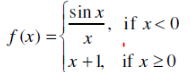Solution
This function f is defined at all points of the real line.
For continuity in these type of question, we should look for the continuity at the common point and point outside the common point
Let c be a point on a real line. Then, c < 0 or c = 0 or  c> 0
It can be easily proved for c < 0 And c> 0. We have seen that in lot of previous example, we will look at common points
At c=0
f(0) = 0+1=1
The left-hand limit of f at x = 0 is,
$\lim_{x \rightarrow 0^-} f(x) =\lim_{x \rightarrow 0^-} \frac {sin x}{x} = 1$
The right-hand limit of f at x = 0 is,
$\lim_{x \rightarrow 0^+} f(x) =\lim_{x \rightarrow 0^+} (x+1) = 1$
Therefore, f is continuous at x = 1
Hence, There is no point of discontinuity of f.

Question 24.
Determine if f defined by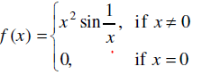is a continuous function?
Solution
This function f is defined at all points of the real line.
For continuity in these type of question, we must look for the continuity at the common point and point outside the common point
Let c be a point on a real line. Then, c < 0 or c = 0 or   c> 0
It can be easily proved for c < 0 And c> 0. We have seen that in lot of previous example, we will look at common points
At c=0
f(0) = 0
The left-hand limit of f at x = 0 is,
$\lim_{x \rightarrow 0^-} f(x) =\lim_{x \rightarrow 0^-} \frac x^2$ Now we know that, for x≠0
$-1 \leq sin \frac {1}{x} \leq 1$
Multiplying by x2
$-x^2 \leq x^2sin \frac {1}{x} \leq x^2$
$\lim_{x \rightarrow 0^-} -x^2 \leq \lim_{x \rightarrow 0^-} x^2sin \frac {1}{x} \leq \lim_{x \rightarrow 0^-} x^2$
Or
$0 \leq \lim_{x \rightarrow 0^-} x^2sin \frac {1}{x} \leq 0$
So
$\lim_{x \rightarrow 0^-} x^2sin \frac {1}{x}=0$
Similarly   The right-hand limit of f at x = 0 can be proved
$\lim_{x \rightarrow 0^+} x^2sin \frac {1}{x}=0$
Therefore, f is continuous at x = 0
Hence, There is no point of discontinuity of f.

Question 25.
Examine the continuity of f, where f is defined by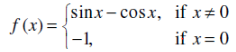Solution
This function f is defined at all points of the real line.
For continuity in these type of question, we must look for the continuity at the common point and point outside the common point
Let c be a point on a real line. Then, c < 0 or c = 0 or   c> 0
It can be easily proved for c < 0 And c> 0. We have seen that in lot of previous example, we will look at common points
At c=0
f(0)=-1
The left-hand limit of f at x = 0 is,
$\lim_{x \rightarrow 0^-} f(x) =\lim_{x \rightarrow 0^-} sin x -cos x = -1$
The right-hand limit of f at x = 0 is,
$\lim_{x \rightarrow 0^+} f(x) =\lim_{x \rightarrow 0^+} sin x -cos x = -1$
Therefore, f is continuous at x = 0
From the above observations, it can be concluded that f is continuous at every point of the real line.
Thus, f is a continuous function.

### Find the values of k so that the function f is continuous at the indicated point in Exercises 26 to 29.

Question 26.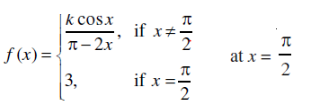Solution
For the function to be continuous at x=π/2, we have
$f(\pi/2) = \lim_{x \rightarrow /pi/2} f(x)$
F(π/2) =3
$\lim_{x \rightarrow /pi/2} f(x)=\lim_{x \rightarrow /pi/2} \frac {kcosx}{\pi -2x}$
Let x = π/2 + h
When x → π/2, then h → 0
$\lim_{x \rightarrow /pi/2} f(x)= \lim_{h \rightarrow 0}\frac {kcos(\pi/2 + h}{\pi -2(\pi/2 +h)}$
$=\lim_{h \rightarrow 0} \frac{-k sinh}{-2h}= \frac {k}{2} \lim_{h \rightarrow 0} \frac {sin h}{h}= \frac {k}{2}$
Now k/2=3
K=6

Question 27.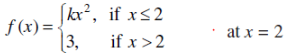Solution
For the function to be continuous at x=2, we have
$\lim_{x \rightarrow 2} f(x) = f(2)$ F(2) =4k
The left-hand limit of f at x = 2 is,
$\lim_{x \rightarrow 2^-} f(x)=4k$
The right-hand limit of f at x = 2 is,
$\lim_{x \rightarrow 2^+} f(x)=3$
Now
4k=4k=3
Or
K=3/4

Question 28.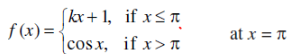Solution
For the function to be continuous at x=π, we have
$f(\pi)=\lim_{x \rightarrow \pi} f(x)$
F(π) =k π+1
The left-hand limit of f at x = π is,
$\lim_{x \rightarrow \pi^-} f(x)=\lim_{x \rightarrow \pi^-} (kx+1)=\pi k + 1$
The right-hand limit of f at x = π  is,
$\lim_{x \rightarrow \pi^+} f(x)=\lim_{x \rightarrow \pi^+} (cos x)=-1$
Now
k π+1=-1
k=-2/ π

Question 29.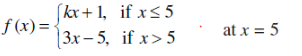Solution
For the function to be continuous at x=5, we have
$\lim_{x \rightarrow 5} f(x)= f(5)$ F(5) =5k+1
The left-hand limit of f at x = 5 is,
$\lim_{x \rightarrow 5^-} f(x)=\lim_{x \rightarrow 5^-} (kx+1)=5 k + 1$
The right-hand limit of f at x = 5  is,
$\lim_{x \rightarrow 5^+} f(x)=\lim_{x \rightarrow 5^+} (3x-5)=10$
Now
5k+1=10
k=9/5

Question 30.
Find the values of a and b such that the function defined by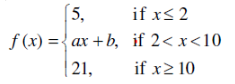is a continuous function.
Solution
The function is defined for all the real values and it is given as continuous
It is also continuous at x=2 and x=10
Let’s check the continuity at those values
At x=2
f(2)=5
The left-hand limit of f at x = 2 is,
$\lim_{x \rightarrow 2^-} f(x)=\lim_{x \rightarrow 2^-} 5=5$
The right-hand limit of f at x = 2  is,
$\lim_{x \rightarrow 2^+} f(x)=\lim_{x \rightarrow 2^+} (ax+b)=2a + b$
Now
2a+b=5  -(P)
At x=10
F(10)=21
The left-hand limit of f at x = 10 is,
$\lim_{x \rightarrow 10^-} f(x)=\lim_{x \rightarrow 10^-} (ax+b)=10a + b$
The right-hand limit of f at x = 10 is,
$\lim_{x \rightarrow 10^+} f(x)=\lim_{x \rightarrow 10^+} 21=21$
Now
10a+b=21 --(Q)
From (p) and (q)
a=2 and b=1

Question 31.
Show that the function defined by f (x) = cos (x2) is a continuous function.
Solution
This function f is defined for every real number and f can be written as the composition of two functions, as,
f = g o h, where g (x) = cos x and h (x) = x2
Because g o h =g[h(x)] =cos (x2)
Now It is known that for real valued functions g and h, such that (g o h) is defined at c, if g is
continuous at c and if f is continuous at g (c), then (f o g) is continuous at c.
So, we just need to prove that g(x) =cos(x) and h(x) =x2 are continuous function
First let us prove for g(x)=cos (x)
It is evident that g (x) = cos x is defined for every real number.
g(c) =cos(c)
Let c be a real number. Put x = c + h
If x → c, then h → 0
$\lim_{x \rightarrow c} g(x) =\lim_{x \rightarrow c} cos x$
$=\lim_{h \rightarrow 0} cos(c+h)=\lim_{h \rightarrow 0} [cos c cos h - sin c sin h]$
$=\lim_{h \rightarrow 0} cos c cos h + \lim_{h \rightarrow 0} sin c sin h= cos c + 0 =cos c$
So, g(x) is continuous
Now h (x) = x2
It is evident that h (x) = x2 is defined for every real number.
h(c) =c2
$\lim_{x \rightarrow c} h(x) =\lim_{x \rightarrow c} x^2=c^2$
So, h(x) is continuous
Since both g(x) and h(x) are continuous. F(x) is continuous function

Question 32.
Show that the function defined by f(x) = | cos x | is a continuous function.
Solution
This function f is defined for every real number and f can be written as the composition
of two functions, as,
f = g o h, where g (x) = |x| and h (x) = cos x
Because g o h =g[h(x)] =| cos x |
Now It is known that for real valued functions g and h, such that (g o h) is defined at c, if g is
continuous at c and if f is continuous at g (c), then (f o g) is continuous at c.
So, we just need to prove that g(x) =|x| and h(x) =cos(x) are continuous function
First let us prove for g(x)=|x|
It can be re-written as
g(x) = -x   for x < 0
=+x for x ≥0
It is evident that g (x) is defined for every real number.
For c < 0
g(c) =-c
$\lim_{x \rightarrow c} g(x) =\lim_{x \rightarrow c} -x=-c$
So, it is continuous
For c = 0
g(c) =0
$\lim_{x \rightarrow 0^-} g(x) =\lim_{x \rightarrow 0^-} -x=0$
$\lim_{x \rightarrow 0^+} g(x) =\lim_{x \rightarrow 0^+} x=0$
So, it is continuous
For c > 0
g(c) =c
$\lim_{x \rightarrow c} g(x) =\lim_{x \rightarrow c} x=c$
So, it is continuous
let us prove for h(x)=cos (x)
It is evident that h (x) = cos x is defined for every real number.
h(c) =cos(c)
Let c be a real number. Put x = c + h
If x → c, then h → 0
$\lim_{x \rightarrow c} h(x) =\lim_{x \rightarrow c} cos x$
$=\lim_{h \rightarrow 0} cos(c+h)=\lim_{h \rightarrow 0} [cos c cos h - sin c sin h]$
$=\lim_{h \rightarrow 0} cos c cos h + \lim_{h \rightarrow 0} sin c sin h= cos c + 0 =cos c$
So, h(x) is continuous
Since both g(x) and h(x) are continuous. F(x) is continuous function

Question 33.
Examine that sin | x | is a continuous function.
Solution
This function f is defined for every real number and f can be written as the composition
of two functions, as,
f = g o h, where g (x) = sin(x) and h (x) = |x|
Because g o h =g[h(x)] = sin | x |
Now It is known that for real valued functions g and h, such that (g o h) is defined at c, if g is
continuous at c and if f is continuous at g (c), then (f o g) is continuous at c.
So, we just need to prove that g(x) =sin (x) and h(x) =|x| are continuous function
Let g (x) = sin x
It is evident that g (x) = sin x is defined for every real number.
g(c) =sin(c)
Let c be a real number. Put x = c + h
If x → c, then h → 0
$\lim_{x \rightarrow c} g(x) =\lim_{x \rightarrow c} sinx$
$=\lim_{h \rightarrow 0} sin(c+h)=\lim_{h \rightarrow 0} [sin c cos h + cos c sin h]$
$=\lim_{h \rightarrow 0} sin c cos h + \lim_{h \rightarrow 0} cos c sin h= sin c + 0 =sin c$
So, g(x) is continuous function
So, g(x) is continuous function
Now let us prove for h(x)=|x|
It can be re-written as
h(x) = -x   for x < 0
=+x for x ≥0
It is evident that h (x) is defined for every real number.
For c < 0
h(c) =-c
$\lim_{x \rightarrow c} h(x) =\lim_{x \rightarrow c} -x=-c$
So, it is continuous
For c = 0
h(c) =0
$\lim_{x \rightarrow 0^-} h(x) =\lim_{x \rightarrow 0^-} -x=0$
$\lim_{x \rightarrow 0^+} h(x) =\lim_{x \rightarrow 0^+} x=0$
So, it is continuous
For c > 0
h(c) =c
$\lim_{x \rightarrow c} h(x) =\lim_{x \rightarrow c} x=-c$
So, it is continuous
So h(x) is continous
Since both g(x) and h(x) are continuous. F(x) is continuous function

Question 34.
Find all the points of discontinuity of f defined by f (x) = | x | – |x + 1|.
Solution
The two functions, g and h, are defined as
g(x) =|x|
h(x) =|x+1|
Then, f = g – h
It is known that if g and h are two continuous functions, then g + h,g − h,g.h are also continuous functions.
So, we just need to prove that g(x) and h(x) is continuous
The continuity of g and h is examined first.
First let us prove for g(x)=|x|
It can be re-written as
g(x) = -x   for x < 0
=+x for x ≥0
It is evident that g (x)  is defined for every real number.
For c < 0
g(c) =-c
$\lim_{x \rightarrow c} g(x) =\lim_{x \rightarrow c} -x=-c$
So, it is continuous
For c = 0
g(c) =0
$\lim_{x \rightarrow 0^-} g(x) =\lim_{x \rightarrow 0^-} -x=0$
$\lim_{x \rightarrow 0^+} g(x) =\lim_{x \rightarrow 0^+} x=0$
So, it is continuous
For c > 0
g(c) =c
$\lim_{x \rightarrow c} g(x) =\lim_{x \rightarrow c} x=-c$
So, it is continuous
Now let us look
h(x)= |x+1|
It can be re-written as
h(x) = -(x+1)   for x < -1
=x+1 for x ≥-1
It is evident that h (x) is defined for every real number.
For c < -1
h(c) =-(c+1)
$\lim_{x \rightarrow c} h(x) =\lim_{x \rightarrow c} -(x+1)=-(c+1)$
So, it is continuous
For c = -1
h(-1) =0
$\lim_{x \rightarrow -1^-} h(x) =\lim_{x \rightarrow -1^-} -(x+1)=0$
$\lim_{x \rightarrow -1^+} h(x) =\lim_{x \rightarrow -1^+} (x+1)=0$
So it is continuous
For c > -1
h(c) =c+1
$\lim_{x \rightarrow c} h(x) =\lim_{x \rightarrow c} (x+1)=(c+1)$
So, it is continuous
So, both g(x) and h(x) are continuous
Therefore, f = g − h is also a continuous function.
Therefore, f has no point of discontinuity.

Go back to Class 12 Main Page using below links